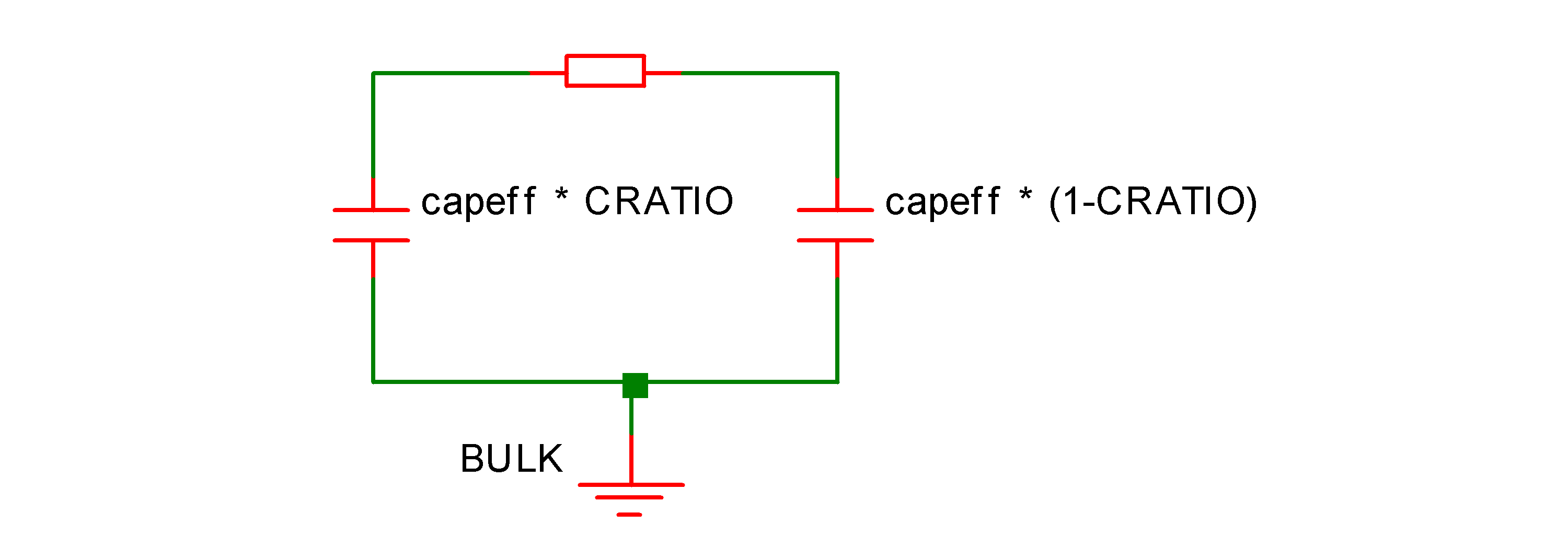# Resistor - Hspice Compatible

In this topic:

## Netlist Entry

Rxxxx n1 n2 [model_name] [value] [L=length] [W=width] [AC=ac_resistance] [TC1=tc1]
[TC2=tc2] [M=mult] [SCALE=scale] [DTEMP=dtemp] [C=c]
 model_name Name of .MODEL. This is compulsory unless this model made the default resistor. See Making the Hspice Resistor the Default. value Value of resistor. This may be an expression relating parameters defined using .PARAM and circuit variables in the form V(n1,n2) and I(device). Expressions must be enclosed in curly braces or quotation marks. Typically the expression relates to the resistor's own terminals in order to define voltage dependence length Length in metres width Width in metres ac_resistance Value of resistor for AC analyses. Like the main value, this may also be an expression. See above under value for details tc1 First order temperature coefficient tc2 Second order temperature coefficient mult Device multiplier. Equivalent to putting mult devices in parallel, but note that this value does not have to be integral scale Scales resistance and capacitance values. E.g. Reff=scale*R dtemp Differential temperature. Device temperature = global temperature + dtemp c Capacitance to reference node

## Resistor Model Syntax

.model modelname R (LEVEL=2 parameters )
 Name Description Units Default BULK Bulk connection for capacitance Ground CAP Device capacitance F 0.0 CAPSW Sidewall capacitance F/m 0.0 COX Capacitance per unit area F/m2 0.0 CRATIO Capacitance terminal distribution 0.5 DI Dielectric constant 0.0 DL Difference between drawn length and actual length for capacitance calculation m DW DLR Difference between drawn length and actual length for resistance calculation m 0.0 DW Difference between drawn width and actual width m 0.0 L, ML Length m 0.0 W, MW Width m 0.0 NOISE Noise multiplier 1.0 RAC AC resistance Ω RES Resistance Ω 0.0 RSH Sheet resistance Ω/sq 0.0 SHRINK Shrink factor 1.0 TC1C Capacitance first order temperature coefficient 1/C 0.0 TC1R Resistance first order temperature coefficient 1/C 0.0 TC2C Capacitance second order temperature coefficient 1/C2 0.0 TC2R Resistance second order temperature coefficient 1/C2 0.0 THICK Dielectric thickness m 0.0 TREF Measurement temperature C TNOM ACRESMOD AC resistance model selector. See ACRESMOD Parameter 0 KF Flicker noise coefficient 0 AF Flicker noise current exponent 2.0 LF Flicker noise length exponent 1.0 WF Flicker noise width exponent 1.0 EF Flicker noise frequency exponent 1.0

## Resistance Calculation

In the following reff is the effective non-temperature adjusted resistance used for DC analyses, reffac is the effective non-temperature adjusted resistance used for AC analyses.

If instance resistance is specified:

reff = value * SCALE/M
otherwise if weff*leff*RSH>0
reff = SCALE*RSH*leff / (weff*M)
where:
weff = SHRINK*W - 2*DW
leff = SHRINK*L - 2*DLR
otherwise
reff = SCALE*RES/M
If instance AC parameter is specified:
raceff = AC*SCALE/M
otherwise if RAC given
raceff = SCALE*RAC/M
otherwise
raceff = reff

## Capacitance Calculationcapeff is the non temperature adjusted capacitance.

If instance parameter C is given:

capeff = M*SCALE*C
otherwise if model parameter CAP is given
capeff = M*SCALE*CAP
otherwise
capeff = (leffc*weffc*coxmod + 2*((leffc+weffc)*CAPSW))*M*SCALE
where:
leffc = SHRINK*L - 2*DL
weffc = SHRINK*W - 2*DW
See below for coxmod calculation.

### Calculation of COX

If COX given

coxmod = COX
otherwise if THICK <> 0 AND DI<>0
coxmod = 8.8542149e-012*DI/THICK
otherwise if THICK <> 0 AND DI=0
coxmod = 3.453148e-011/THICK
otherwise
coxmod = 0.0

## Temperature Scaling

### Resistance

R(t) = reff * tscale
where:
tscale = 1+(tc1inst+tdelta*tc2inst)*tdelta
where
tdelta = tinst-TREF-273.15
tc1inst = if instance TC1 given: TC1 else TC1R
tc2inst = if instance TC2 given: TC2 else TC2R
tinst = [global circuit temperature] + DTEMP (Kelvin)

### Capacitance

C(t) = capeff * tscale
where
tcscale = 1+(TC1C+tdelta*TC2C)*tdelta
tdelta defined above.

## Flicker Noise

$I_n = \frac{\text{KF} \times I^{\text{AF}}}{L_{\text{eff}}^{\text{LF}} \times W_{\text{eff}}^{\text{WF}} \times f^{\text{EF}}}$

## ACRESMOD Parameter

This parameter controls the calculation of resistance in AC analysis. With ACRESMOD=0 AC analysis uses the large signal resistance value, that is the value of resistance calculated during the DC analysis. If ACRESMOD=1, the small signal resistance is used, that is, the value of dv/di at the operating point. If the resistance is defined as an expression containing circuit variables (i.e. it is voltage dependent), the large signal resistance is different to the small signal resistance.

This resistor model has been developed primarily for compatibility with Hspice models. Hspice itself always uses the large signal resistance. However, this will create a discrepancy between AC analysis and a transient analysis of a small signal. To resolve this discrepancy, set ACRESMOD to 1.

In summary, to be compatible with Hspice, use ACRESMOD=0, for consistent results between AC and transient analyses, use ACRESMOD=1.

In noise analysis the large signal value is always used.

## Making the Hspice Resistor the Default

This resistor model requires the specification of a model name and the creation of a .MODEL statement with LEVEL=2. This is likely to be inconvenient if a model file containing Hspice resistors is being used.

To overcome this, the HSPICEMODELS option setting may be used to make this resistor the default. Add this line to the netlist:

.OPTIONS HSPICEMODELS=1
This setting also has the same effect:
.OPTIONS HSPICECOMPATIBILITY=1

See .OPTIONS for more details